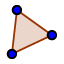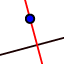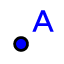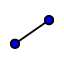Perpendicular Bisector with Circumcenter

Directions

1) Use the POLYGON toolto construct triangles A(2,3) B(6,13) and C(14,3) 2) Use the INTERSECTION toolto construct the Perpendicular Bisector of each side 3) Use the POINT toolto construct the intersection of the 3 Perpendicular Bisectors and record its coordinates on your worksheet. 4) Use the SEGMENT toolto construct the segment from the circumcenter to the vertices.

Questions

1) Is it ever possible for a triangle's circumcenter to lie OUTSIDE the triangle? If so, under what circumstance(s) will this occur? 2) Is it ever possible for a triangle'scircumcenterto lie ON THE TRIANGLE ITSELF? If so, under what circumstance(s) will this occur? 3) If your answer for (2) was "YES", where on the triangle did Circumcenter lie? 4) Is it ever possible for a triangle'scircumcenterto lie INSIDE the triangle? If so, under what circumstance(s) will this occur?